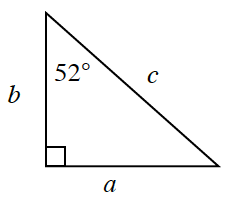### Home > CCG > Chapter 5 > Lesson 5.2.2 > Problem5-66

5-66.Using the triangle below, write an expression representing $\cos52º$. Then write an expression for $\tan52º$ and $\cos38º$. What other ratio is equivalent to $\cos38º$?

Recall that $\cos=\frac{\text{adjacent}}{\text{hypotenuse}}$.

Where is the $38º$ angle in the triangle?

Refer to the Math Notes box in Lesson 5.1.2 for the different trigonometric ratios.